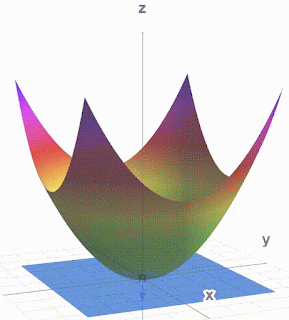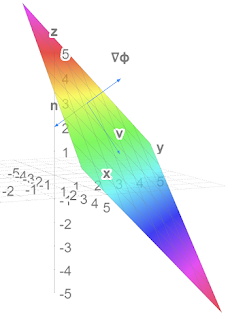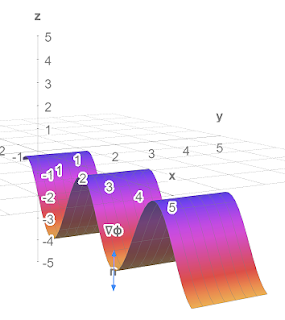## Monday 22 November 2021

### 3D-grapher at math3d.org

I was practicing my tensor analysis and calculated a normal vector to a surface. I wanted to see it looked orthogonal to the surface. It didn't really. So I made a tangent plane to the surface. The normal vector still looks a bit un-orthogonal but it passes all the mathematical tests.Paraboloid, Normal vector and tangent plane $\phi_2\left(\vec{x}\right)=\left(x^1\right)^2+\left(x^2\right)^2-x^3=0$

You can play with this at and stop it from moving.
I also used Debut Video Capture to make a .avi from math3d and then cloudconvert.com to make the .gif. It is simpler than ezgif.com.

Other surfaces and vectors:Plane and normal vectors (not v)Play at https://www.math3d.org/fq5CtGqYa$\phi_1\left(\vec{x}\right)=x^1+x^2+x^3=5$Sine wave with normal vectors Play at https://www.math3d.org/D8xf0gZMT$\phi_3\left(\vec{x}\right)=x^3+r_0\cos{\left(kx^1\right)}=-4$

Lots more tools on Physics Forums at

#### 1 comment:

1.Learning math may not be as simple as it sounds. As we all know Mathematics plays a major role in our life as it is necessary to be accurate in the accounts to maintain money in an optimal manner. From primary schools to colleges mathematics will be tough because it is the main subject. Now Doubt, Math is difficult to study but nowadays many tuitions are available which can help students to crack the exams and the entrance. One of my friends shared with me about online math tuition. which is the best tuition at any level. I hope this will be a great help to many students who really understand the importance of mathematics.